Chapter 5, Problem 5.8EP### Organic And Biological Chemistry

7th Edition
STOKER + 1 other
ISBN: 9781305081079

#### Solutions

Chapter
Section### Organic And Biological Chemistry

7th Edition
STOKER + 1 other
ISBN: 9781305081079
Textbook Problem

# For each of the following type of compounds, all of which have the general formula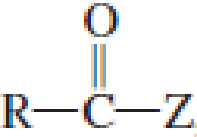, indicate whether the “C─Z bond” is polar or nonpolar.a.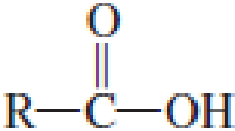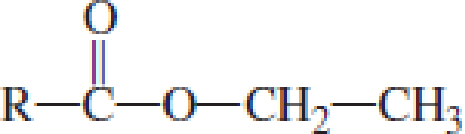(a)

Interpretation Introduction

Interpretation:

The “CZ” bond in the general formula of carboxylic derivative is polar or nonpolar has to be indicated.

Concept Introduction:

Carbonyl groups are the one which contain a double bond between carbon and oxygen atom.  Aldehydes and ketones possess this carbonyl functional group in it.  The structural representation of a carbonyl group can be given as shown below,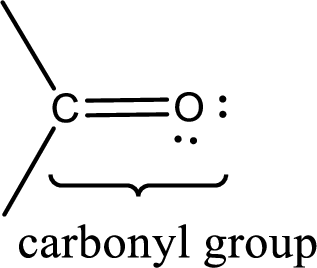If a hydroxyl group is attached to a carbonyl group means it is known as carboxyl group.  This can be represented as shown below,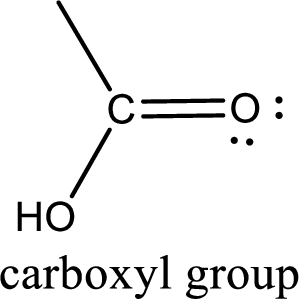Carboxylic acid derivatives are the ones that are synthesized from or converted to a carboxylic acid.  The generalized structural representation of carboxylic acid derivatives is shown below,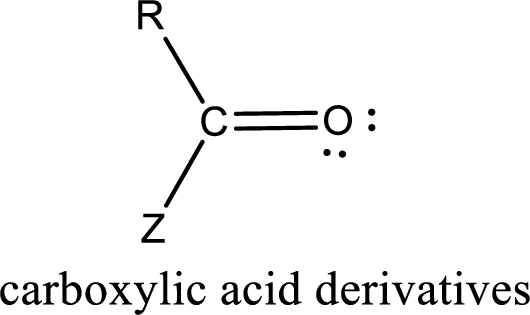In the carboxylic acid derivatives, if the carbonyl carbon atom is bonded to a more electronegative atom means, then the bond will be polar and is it is bonded to carbon atom means then it will be nonpolar.

Explanation

The general structure of carboxylic acid is,

The atom in entity Z that is bonded to the carbonyl carbon atom is not a carbon atom.  It is an oxygen atom...

(b)

Interpretation Introduction

Interpretation:

The “CZ” bond in the general formula of carboxylic derivative is polar or nonpolar has to be indicated.

Concept Introduction:

Carbonyl groups are the one which contain a double bond between carbon and oxygen atom.  Aldehydes and ketones possess this carbonyl functional group in it.  The structural representation of a carbonyl group can be given as shown below,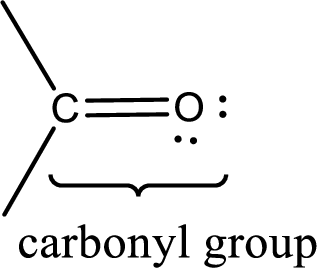If a hydroxyl group is attached to a carbonyl group means it is known as carboxyl group.  This can be represented as shown below,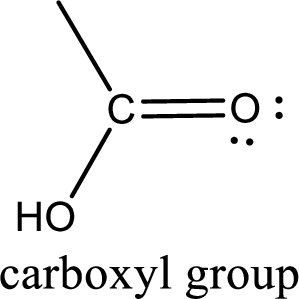Carboxylic acid derivatives are the ones that are synthesized from or converted to a carboxylic acid.  The generalized structural representation of carboxylic acid derivatives is shown below,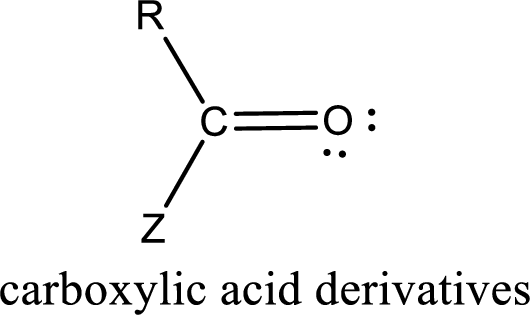In the carboxylic acid derivatives, if the carbonyl carbon atom is bonded to a more electronegative atom means, then the bond will be polar and is it is bonded to carbon atom means then it will be nonpolar.

(c)

Interpretation Introduction

Interpretation:

The “CZ” bond in the general formula of carboxylic derivative is polar or nonpolar has to be indicated.

Concept Introduction:

Carbonyl groups are the one which contain a double bond between carbon and oxygen atom.  Aldehydes and ketones possess this carbonyl functional group in it.  The structural representation of a carbonyl group can be given as shown below,If a hydroxyl group is attached to a carbonyl group means it is known as carboxyl group.  This can be represented as shown below,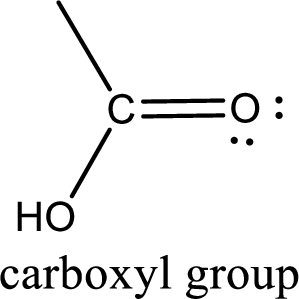Carboxylic acid derivatives are the ones that are synthesized from or converted to a carboxylic acid.  The generalized structural representation of carboxylic acid derivatives is shown below,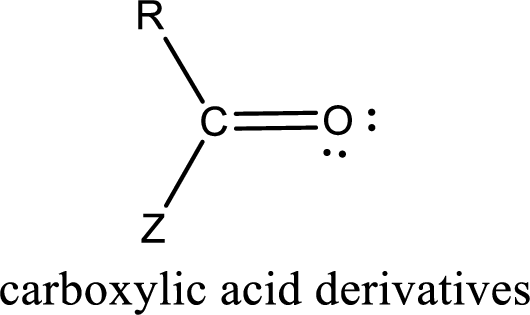In the carboxylic acid derivatives, if the carbonyl carbon atom is bonded to a more electronegative atom means, then the bond will be polar and is it is bonded to carbon atom means then it will be nonpolar.

(d)

Interpretation Introduction

Interpretation:

The “CZ” bond in the general formula of carboxylic derivative is polar or nonpolar has to be indicated.

Concept Introduction:

Carbonyl groups are the one which contain a double bond between carbon and oxygen atom.  Aldehydes and ketones possess this carbonyl functional group in it.  The structural representation of a carbonyl group can be given as shown below,If a hydroxyl group is attached to a carbonyl group means it is known as carboxyl group.  This can be represented as shown below,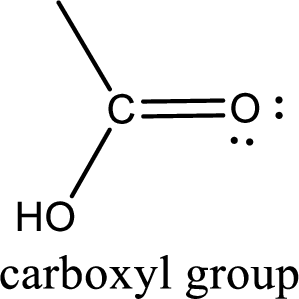Carboxylic acid derivatives are the ones that are synthesized from or converted to a carboxylic acid.  The generalized structural representation of carboxylic acid derivatives is shown below,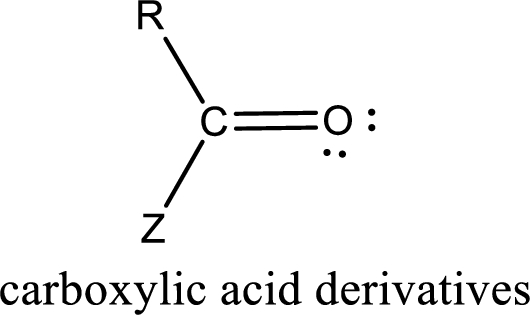In the carboxylic acid derivatives, if the carbonyl carbon atom is bonded to a more electronegative atom means, then the bond will be polar and is it is bonded to carbon atom means then it will be nonpolar.

### Still sussing out bartleby?

Check out a sample textbook solution.

See a sample solution

#### The Solution to Your Study Problems

Bartleby provides explanations to thousands of textbook problems written by our experts, many with advanced degrees!

Get Started